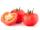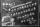Number

Calculate the integer number which, divided by 34 gives 10 and the rest 25.

Result

x =  365

Solution:Leave us a comment of this math problem and its solution (i.e. if it is still somewhat unclear...):Be the first to comment!To solve this verbal math problem are needed these knowledge from mathematics:

Do you have a linear equation or system of equations and looking for its solution? Or do you have quadratic equation?

Next similar math problems:

1. 15 numberWhat number is smaller (greater) by 15 than its half?
2. Double 5Peter was thinking of a number. Peter doubles it and gets an answer of 8.6. What was the original number?
3. What isWhat is the value of the smaller of a pair of numbers for which their sum is 78 and their division quotients are 0.3?
4. Two numbers 6Fill two natural numbers a, b: 7 + blank- blank = 5
5. EquatiomSolve equation with negatives: X/(-5) + 2 = -9
6. Fractions 4How many 2/3s are in 6?
7. Farmers 2On Wednesday the farmers at the Grant Farm picked 2 barrels of tomatoes. Thursday, the farmers picked 1/2 as many tomatoes as on Wednesday. How many barrels of tomatoes did the farmers pick on Thursday?
8. Scouts 44/7 of the students in a school are boys. If 3/8 of the boys are scouts, how many scouts are there in a school of 1878 students?
9. Simple equation 8Solve the following equation: 36=-(1+7x)-6(-7-x)
10. Forest nurseryIn the forest nursery after winter, they found that 1/10 stems died out of them. For them, they land 193 new spruces. How many spruces are in the forest nursery?
11. Simple equation 9Solve the following equation: -8y+5=-9y+9
12. Six te 2If 3t-7=5t, then 6t=
13. Negative in equation2x + 3 + 7x = – 24, what is the value of x?
14. Find xSolve: if 2(x-1)=14, then x= (solve an equation with one unknown)
15. Equation 29Solve next equation: 2 ( 2x + 3 ) = 8 ( 1 - x) -5 ( x -2 )
16. Simple equation 5Solve equation with fractions: X × 3/8 = 1/2
17. Equation 20In given equation: 8/9-4/5=2/9+x, find x